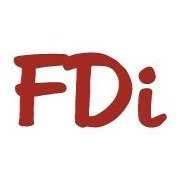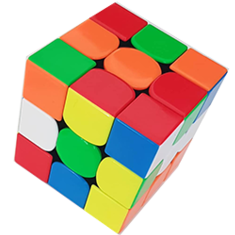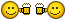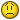# Convert polyline with arc to polyline with straight lines.## Recommended PostsHi all - I created a lightweight polyline by filleting two straight polylines to create 1 polyline that contains an arc. I would like to convert the arc into a series of straight polylines that mimic the original shape of the arc, while not disturbing the straight parts of the polyline. I found a .lsp routine that does this perfectly, but it only works for the older heavyweight polylines. Can anyone help modify this routine so it works for lightweight polylines or provide another suggestion? Id rather not convert to heavyweight, use the routine, and convert back to lightweight. Thanks so much!

Here is the code:

```;;;Translate plines w/ arcs to plines w/ mult. straight segments for use
;;;with DTM TIN's for contouring.  Rounds out pline arcs via a suitable no.
;;;of straight segments.  The determiner is based on small angle deflection.
;;;
;;;	AUTHOR: HENRY C. FRANCIS
;;;		425 N. ASHE ST.
;;;		SOUTHERN PINES, NC 28387
;;;
;;;
;;;	Edited:		10-1-98
;;;
(DEFUN c:plxl (/ found)
(SETQ	osmod (GETVAR "osmode")
fltot 0
incrn 0
) ;_ end of setq
(SETVAR "osmode" 0)
(SETQ	usrdeg (ureal 1
""
"Deflection angle (< 5 degrees)"
(IF usrdeg
usrdeg
5.0
) ;_ end of if
) ;_ end of ureal
) ;_ end of setq
(SETQ usrrad (* (/ usrdeg 180.0000) PI))
(SETQ pliness (SSGET '((0 . "POLYLINE"))))
(IF pliness
(PROGN
(COMMAND ".undo" "m")
(SETQ plinesslen
(SSLENGTH pliness)
sscount 0
) ;_ end of setq
(WHILE (< sscount plinesslen)
(SETQ currpline (SSNAME pliness sscount))
(SETQ plent (ENTGET currpline))
(SETQ plvert (ENTGET (ENTNEXT (CDAR plent))))
(PROGN
(ENTMAKE
(LIST
(ASSOC 0 plent)
(ASSOC 8 plent)
(ASSOC 66 plent)
(ASSOC 10 plent)
(ASSOC 70 plvert)
) ;_ end of list
) ;_ end of entmake
(ENTMAKE
(LIST
(ASSOC 0 plvert)
(ASSOC 10 plvert)
) ;_ end of list
) ;_ end of entmake
;;;----repeat this until the end of the polyline
(WHILE (/= (CDR (ASSOC 0 (ENTGET (ENTNEXT (CDAR plvert)))))
"SEQEND"
) ;_ end of /=
;;;------if it begins an arc segment
(IF	(/= (CDR (ASSOC 42 plvert)) 0)
;;;--------do this
(PROGN
(SETQ found T)
(SETQ plnvert (ENTGET (ENTNEXT (CDAR plvert))))
(SETQ strt40 (CDR (ASSOC 40 plvert)))
(SETQ end41 (CDR (ASSOC 41 plvert)))
(SETQ fpt1 (CDR (ASSOC 10 plvert)))
(SETQ fpt2 (CDR (ASSOC 10 plnvert)))
(SETQ chrdl (DISTANCE fpt1 fpt2))
(SETQ theta (ATAN (CDR (ASSOC 42 plvert))))
(SETQ psi (- (/ PI 2) (ABS theta)))
(SETQ phi (* (ABS theta) 4))
(SETQ chang (ANGLE fpt1 fpt2))
(SETQ arcr (ABS	(/ (* (DISTANCE fpt1 fpt2) (SIN psi))
(* 2 (COS theta) (SIN (* 2 theta)))
) ;_ end of /
) ;_ end of abs
) ;_ end of setq
(SETQ arcc
(IF (> theta 0)
(POLAR fpt1 (+ (- chang theta) psi) arcr)
(POLAR fpt1 (- (- chang theta) psi) arcr)
) ;_ end of if
) ;_ end of setq
(SETQ fenl    (* phi arcr)
count   (1+ (FIX (/ phi usrrad)))
plwinc  (/ (- strt40 end41) count)
plwe    (+ strt40 plwinc)
incra   (/ phi count)
incrn   0
initang (ANGLE arcc fpt1)
) ;_ end of setq
(WHILE
(> count 0)
(SETQ incrn (1+ incrn))
(SETQ plwb plwe
plwe (- plwe plwinc)
) ;_ end of setq
(IF (< theta 0)
(SETQ fpt4
(POLAR arcc (- initang (* incrn incra)) arcr)
) ;_ end of setq
(SETQ fpt4
(POLAR arcc (+ initang (* incrn incra)) arcr)
) ;_ end of setq
) ;_ end of if
(PROGN
(ENTMAKE
(LIST
(CONS 0 "VERTEX")
(ASSOC 8 plvert)
(CONS 10 fpt4)
) ;_ end of list
) ;_ end of entmake
(GRDRAW fpt1 fpt4 -1)
) ;_ end of progn
(SETQ fpt1  fpt4
count (1- count)
) ;_ end of setq
) ;_ end of while
(SETQ plvert (ENTGET (ENTNEXT (CDAR plvert))))
) ;_ end of progn
;;;--------or else it begins a line segment so do this
(PROGN
(SETQ fpt1 (CDR (ASSOC 10 plvert)))
(SETQ fpt2
(CDR (ASSOC 10 (ENTGET (ENTNEXT (CDAR plvert)))))
) ;_ end of setq
(SETQ fenl (DISTANCE fpt1 fpt2))
(ENTMAKE
(LIST
(CONS 0 "VERTEX")
(ASSOC 8 plvert)
(CONS 10 fpt2)
) ;_ end of list
) ;_ end of entmake
(GRDRAW fpt1 fpt2 -1)
(SETQ fpt1 fpt2)
(SETQ plvert (ENTGET (ENTNEXT (CDAR plvert))))
) ;_ end of progn
) ;_ end of if
) ;_ end of while
(IF found
(PROGN
(ENTMAKE
(LIST
(CONS 0 "SEQEND")
) ;_ end of list
) ;_ end of entmake
(ENTDEL currpline)
) ;_ end of progn
(PROGN
(ENTMAKE)
(COMMAND ".redraw")
(PRINC "\nPolyline contains no arcs. ")
) ;_ end of progn
) ;_ end of if
) ;_ end of progn
(SETQ sscount (1+ sscount))
) ;_ end of WHILE
) ;_ end of progn
) ;_ end of if
(PRINC)
) ;_ end of defun

;|«Visual LISP© Format Options» (72 2 40 2 T "end of " 60 9 2 0 0 T T nil T)
***Don't add text below the comment!***|;```

```;This function is freeware courtesy of the author's of "Inside AutoLisp" for rel. 10 published by New Riders Publications.  This credit must accompany all copies of this function.
;
;* UREAL User interface real function
;* BIT (0 for none) and KWD key word ("" for none) are same as for INITGET.
;* MSG is the prompt string, to which a default real is added as <DEF> (nil
;* for none), and a : is added.
;*
(defun ureal (bit kwd msg def / inp)
(if def
(setq msg (strcat "\n" msg " <" (rtos def 2) ">: ")
bit (* 2 (fix (/ bit 2)))
)
(setq msg (strcat "\n" msg ": "))
);if
(initget bit kwd)
(setq inp (getreal msg))
(if inp inp def)
);defun
;*
(princ)
;*```

##### Share on other sitesI've used lots of variations of this over the years:

ARG -> PLINE ename

RET -> LIST of point values

```;++++++++++++ FINDPATH +++++++++++++++++++++++++++++++++++++++++++
;;;Returns ECS Point Values Of PLINE
(defun findpath (en / pl ed sp_flg cl_flg bf nl i vp bf vf pl_flg)
(if (= "LWPOLYLINE" (cdr (assoc 0 (entget en))))
(command "_.CONVERTPOLY" "_Heavy" en ""))
(setq ed (entget en))
(and (/= "POLYLINE" (cdr (assoc 0 ed)))
(princ "\n*** POLYLINEs Only *** ")
(exit))
(setq pl_flg (cdr (assoc 70 ed)))
(and (= (logand pl_flg 1) 1)
(setq cl_flg T))
(and (= (logand pl_flg 4) 4)
(setq sp_flg T))
(and (or (= (logand pl_flg 16) 16)
(= (logand pl_flg 64) 64))
(princ "\nInvalid POLYLINE Mesh")
(exit))
(while (/= "SEQEND" (cdr (assoc 0 (entget (entnext en)))))
(setq en (entnext en)
ed (entget en)
vp (cdr (assoc 10 ed))
bf (cdr (assoc 42 ed))
vf (cdr (assoc 70 ed)))
(cond ((and (/= bf 0.0)
cl_flg
(= "SEQEND" (cdr (assoc 0 (entget (entnext en))))))
((= "SEQEND" (cdr (assoc 0 (entget (entnext en)))))
(= bf 0.0)
(not cl_flg)
(setq pl (cons vp pl)))
((and (/= bf 0.0)
(/= "SEQEND" (cdr (assoc 0 (entget (entnext en))))))
(add_arc vp (cdr (assoc 10 (entget (entnext en)))) bf))
((and (= bf 1.0)
(not cl_flg)
(= "SEQEND" (cdr (assoc 0 (entget (entnext en))))))
(princ))
((and sp_flg
(= bf 0.0)
(= (logand vf  8))
(setq pl (cons vp pl)))
((and (not sp_flg)
(= bf 0.0)
(/= (logand vf  8))
(setq pl (cons vp pl)))))
(if (and cl_flg
(not (equal (car pl) (last pl))))
(setq pl (cons (last pl) pl)))
(setq i 0)
(while (< i (length pl))
(while (equal (nth i pl) (nth (1+ i) pl) 0.0001)
(setq i (1+ i)))
(and (nth i pl)
(setq nl (cons (nth i pl) nl)))
(setq i (1+ i)))
nl)

(defun add_arc (sp ep bulge / alist x1 x2 y1 y2 cotbce
ce ra sa ea ia inc qty na temp)
(setq x1 (car sp);;Modified Bulge
x2 (car ep);;Conversion By
cotbce (/ (- (/ 1.0 bulge) bulge) 2.0)
ce (list (/ (+ x1 x2 (- (* (- y2 y1) cotbce))) 2.0)
(/ (+ y1 y2	(* (- x2 x1) cotbce) ) 2.0)
ra (distance ce sp)
sa (atan (- y1 (cadr ce)) (- x1 (car ce)))
ea (atan (- y2 (cadr ce)) (- x2 (car ce))))
(if (minusp sa)
(setq sa (+ sa (* 2.0 pi))))
(if (minusp ea)
(setq ea (+ ea (* 2.0 pi))))
(if (minusp bulge)
(setq temp sa sa ea ea temp))
(if (> sa ea)
(setq ia (+ (- (* pi 2.0) sa) ea))
(setq ia (- ea sa)))
(setq qty (max 2 (abs (fix (/ ia (/ pi 16) 2)))));;; SEGMENT QTY
(setq na sa
inc (/ (abs ia) qty))
(repeat (1+ qty)
(setq alist (cons (polar ce na ra) alist)
na (+ sa inc)
sa na))
(if (not (equal sp (car alist) 0.0001))
(setq alist (reverse alist)))
(foreach a alist
(setq pl (cons a pl))))
```

Maybe it will help. -David

##### Share on other sitesThis edited sub routine might help you as well. a sub i used before for modified Xclip

```(defun c:ArcToLine  (/ *error* blg blk ent objts cnt blgLoc pts stp mxp cur
ent2d)
(defun *error* (msg)
(and uFlag (vla-EndUndoMark doc))
(and ov (mapcar (function setvar) vl ov))
(and msg
(or (wcmatch (strcase msg) "*BREAK,*CANCEL*,*EXIT*")
(princ (strcat "\n** Error: " msg " **"))
)
)
(princ)
)
(defun blg (ent num / blg)
(repeat num
(setq blg (cons (list
(vla-getbulge ent (setq num (1- num)))
(trans (vlax-safearray->list
(variant-value
(vla-Get-coordinate ent num)
)
)
0
1
)
)
blg
)
)
)

)
vl  '("CMDECHO" "OSMODE" "ORTHOMODE")
ov  (mapcar (function getvar) vl)
)
(prompt "\nSelect LWPOLYLINE To convert:")
(if
(and
(setq uFlag (not (vla-StartUndoMark doc)))
(mapcar (function setvar) vl '(0 0 0))
(setq pts nil ent (car (entsel "\nSelect Polyline Boundary:\n")))
(eq (cdr (assoc 0 (entget ent))) "LWPOLYLINE")
(setq alen (getdist "\nEnter line ncrement length: "))
)
(progn
(setq objts (vlax-ename->vla-object ent))
(setq cnt    0
blgLoc (blg objts (cdr (assoc 90 (entget ent))))
)
(foreach itm blgLoc
(setq cnt (1+ cnt))
(if (= (car itm) 0.0)
(setq pts (cons (trans (cadr itm) 1 0) pts))
(progn
(setq pts (cons (trans (cadr itm) 1 0) pts))
(setq stp (if (zerop
(setq cur (vlax-curve-getDistAtPoint
objts
)
)
)
(vla-get-length objts)
cur
)
nxp (if (>= (1+ cnt) (cdr (assoc 90 (entget ent))))
(vla-get-length objts)
(vlax-curve-getDistAtPoint
objts
(trans (cadr (nth cnt blgLoc)) 1 0)
)
)
)
(while (< (setq stp (+ stp alen)) nxp)
(setq
pts (cons (vlax-curve-getPointAtDist objts stp) pts)
)
)
)
)
)
clr
(if pts
(progn
(setq
ent2d (entmakex
(append
(list (cons 0 "LWPOLYLINE")
(cons 100 "AcDbEntity")
(cons 100 "AcDbPolyline")
(cons 90 (length pts))
(cons 70 0)
)
(mapcar (function (lambda (p) (cons 10 p))) pts)
)
)
)(entdel ent)
)
)
(setq uFlag (vla-EndUndoMark doc))
)
)
(*error* nil)
(princ)
)```

##### Share on other sitesTry this - used ChNthDxf sub-function from HofCad long time ago...

```(defun ChNthDxf (e n code value / ed newDxf i oldDxfv k)
(setq ed (entget e))
(setq newDxf '())
(setq oldDxfv '())
(setq i 0)
(foreach v ed
(if (= (car v) code)
(progn
(setq i (+ i 1))
(if (= i n)
(progn
(if (= value nil)
(setq oldDxfv (cons v oldDxfv))
(progn
(setq newDxf (cons (cons code value) newDxf))
(setq oldDxfv (cons v oldDxfv))
)
)
)
(setq newDxf (cons v newDxf))
)
)
(setq newDxf (cons v newDxf))
)
)
(foreach v ed
(if (= (car v) code)
(progn
(setq k (+ i 1))
(if (= k n)
(setq newDxf (cons (cons code value) newDxf))
)
)
)
)
(if (not (assoc code ed))
(setq newDxf (cons (cons code value) newDxf))
)

(entmod (reverse newDxf))
(entupd e)
)

(defun c:plstreighten ( / pl vertn k )
(setq pl (car (entsel "\nPick LWPOLYLINE with arcs you want to streighten")))
(setq vertn (cdr (assoc 90 (entget pl))))
(setq k -1)
(repeat vertn
(setq k (1+ k))
(chnthdxf pl k 42 0.0)
)
(princ)
)

(defun c:plsegstreighten ( / entspl pl pt k ) (vl-load-com)
(setq entspl (entsel "\nPick LWPOLYLINE segment with arc you want to streighten"))
(setq pl (car entspl))
(setq k (+ (fix (vlax-curve-getparamatpoint pl (osnap pt "nea"))) 1))
(chnthdxf pl k 42 0.0)
(princ)
)
```

M.R.

Edited by marko_ribar
##### Share on other sitesThanks pBe - i was able to use your routine and get the results i needed.

##### Share on other sitesThanks pBe - i was able to use your routine and get the results i needed.

Cool beans

Cheers##### Share on other sites

• 4 years later...pBe - Thanks for posting this routine - it seems to work great for me except for one thing. It seems that if the original polyline starts with an arc, it just turns that entire arc into 1 straight line instead of tracing it with the desired segment size like the rest of the arcs. I'd love to have a version of this routine that can fixes this behavior. Thanks for any help!

##### Share on other sitespBe - Thanks for posting this routine - it seems to work great for me except for one thing. It seems that if the original polyline starts with an arc, it just turns that entire arc into 1 straight line instead of tracing it with the desired segment size like the rest of the arcs. I'd love to have a version of this routine that can fixes this behavior. Thanks for any help!

This is an old thread, I suggest that you use "lws-arcs-seg-d.lsp" from PLINETOOLS from here :

HTH, M.R.

##### Share on other sitesPL-NOARC.LSP used a set http://dwg.ru/dnl/607

Segment Length given in the line (setq reg 1.0

In the attached drawing the top line to the conversion, the bottom after.

Sorry for bad English.PL-NOARC.LSP

PL-NOARC.dwg

##### Share on other sites

• 2 years later...Hi,

I want to use these subroutines in a routine which writes the Point list to a XML file.

However when I catch the return of the FINDPATH subroutine in a variable (setq lst1 (findpath en)) it looks like it returns the length of the list and not the list it self. But the NL variable in the FINDPATH subroutine prints the coordinates of the point list in the text window and not the length..am I missing something here?

Greetzzz,

Gerben

I've used lots of variations of this over the years:

ARG -> PLINE ename

RET -> LIST of point values

```;++++++++++++ FINDPATH +++++++++++++++++++++++++++++++++++++++++++
;;;Returns ECS Point Values Of PLINE
(defun findpath (en / pl ed sp_flg cl_flg bf nl i vp bf vf pl_flg)
(if (= "LWPOLYLINE" (cdr (assoc 0 (entget en))))
(command "_.CONVERTPOLY" "_Heavy" en ""))
(setq ed (entget en))
(and (/= "POLYLINE" (cdr (assoc 0 ed)))
(princ "\n*** POLYLINEs Only *** ")
(exit))
(setq pl_flg (cdr (assoc 70 ed)))
(and (= (logand pl_flg 1) 1)
(setq cl_flg T))
(and (= (logand pl_flg 4) 4)
(setq sp_flg T))
(and (or (= (logand pl_flg 16) 16)
(= (logand pl_flg 64) 64))
(princ "\nInvalid POLYLINE Mesh")
(exit))
(while (/= "SEQEND" (cdr (assoc 0 (entget (entnext en)))))
(setq en (entnext en)
ed (entget en)
vp (cdr (assoc 10 ed))
bf (cdr (assoc 42 ed))
vf (cdr (assoc 70 ed)))
(cond ((and (/= bf 0.0)
cl_flg
(= "SEQEND" (cdr (assoc 0 (entget (entnext en))))))
((= "SEQEND" (cdr (assoc 0 (entget (entnext en)))))
(= bf 0.0)
(not cl_flg)
(setq pl (cons vp pl)))
((and (/= bf 0.0)
(/= "SEQEND" (cdr (assoc 0 (entget (entnext en))))))
(add_arc vp (cdr (assoc 10 (entget (entnext en)))) bf))
((and (= bf 1.0)
(not cl_flg)
(= "SEQEND" (cdr (assoc 0 (entget (entnext en))))))
(princ))
((and sp_flg
(= bf 0.0)
(= (logand vf  8))
(setq pl (cons vp pl)))
((and (not sp_flg)
(= bf 0.0)
(/= (logand vf  8))
(setq pl (cons vp pl)))))
(if (and cl_flg
(not (equal (car pl) (last pl))))
(setq pl (cons (last pl) pl)))
(setq i 0)
(while (< i (length pl))
(while (equal (nth i pl) (nth (1+ i) pl) 0.0001)
(setq i (1+ i)))
(and (nth i pl)
(setq nl (cons (nth i pl) nl)))
(setq i (1+ i)))
nl)

(defun add_arc (sp ep bulge / alist x1 x2 y1 y2 cotbce
ce ra sa ea ia inc qty na temp)
(setq x1 (car sp);;Modified Bulge
x2 (car ep);;Conversion By
cotbce (/ (- (/ 1.0 bulge) bulge) 2.0)
ce (list (/ (+ x1 x2 (- (* (- y2 y1) cotbce))) 2.0)
(/ (+ y1 y2    (* (- x2 x1) cotbce) ) 2.0)
ra (distance ce sp)
sa (atan (- y1 (cadr ce)) (- x1 (car ce)))
ea (atan (- y2 (cadr ce)) (- x2 (car ce))))
(if (minusp sa)
(setq sa (+ sa (* 2.0 pi))))
(if (minusp ea)
(setq ea (+ ea (* 2.0 pi))))
(if (minusp bulge)
(setq temp sa sa ea ea temp))
(if (> sa ea)
(setq ia (+ (- (* pi 2.0) sa) ea))
(setq ia (- ea sa)))
(setq qty (max 2 (abs (fix (/ ia (/ pi 16) 2)))));;; SEGMENT QTY
(setq na sa
inc (/ (abs ia) qty))
(repeat (1+ qty)
(setq alist (cons (polar ce na ra) alist)
na (+ sa inc)
sa na))
(if (not (equal sp (car alist) 0.0001))
(setq alist (reverse alist)))
(foreach a alist
(setq pl (cons a pl))))
```

Maybe it will help. -David

## Join the conversation

You can post now and register later. If you have an account, sign in now to post with your account.
Note: Your post will require moderator approval before it will be visible.×   Pasted as rich text.   Restore formatting

Only 75 emoji are allowed.